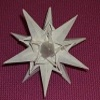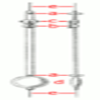Search by Topic

Resources tagged with Differential equations similar to What's My Equation?:

Filter by: Content type:
Age range:
Challenge level:

There are 13 results

Broad Topics > Calculus > Differential equationsWhat's My Equation?

Age 16 to 18 Challenge Level:

Can you find the differential equations giving rise to these famous solutions?It's Only a Minus Sign

Age 16 to 18 Challenge Level:

Solve these differential equations to see how a minus sign can change the answerDifferential Equation Matcher

Age 16 to 18 Challenge Level:

Match the descriptions of physical processes to these differential equations.Bessel's Equation

Age 16 to 18 Challenge Level:

Get further into power series using the fascinating Bessel's equation.The Not-so-simple Pendulum 2

Age 16 to 18 Challenge Level:

Things are roughened up and friction is now added to the approximate simple pendulumTaking Trigonometry Series-ly

Age 16 to 18 Challenge Level:

Look at the advanced way of viewing sin and cos through their power series.Reaction Rates

Age 16 to 18 Challenge Level:

Explore the possibilities for reaction rates versus concentrations with this non-linear differential equationThe Real Hydrogen Atom

Age 16 to 18 Challenge Level:

Dip your toe into the world of quantum mechanics by looking at the Schrodinger equation for hydrogen atomsThe Not-so-simple Pendulum 1

Age 16 to 18 Challenge Level:

See how the motion of the simple pendulum is not-so-simple after all.Modelling Assumptions in Mechanics

Age 16 to 18

An article demonstrating mathematically how various physical modelling assumptions affect the solution to the seemingly simple problem of the projectile.Predator - Prey Systems

Age 16 to 18 Challenge Level:

See how differential equations might be used to make a realistic model of a system containing predators and their prey.Earth Orbit

Age 16 to 18 Challenge Level:

Follow in the steps of Newton and find the path that the earth follows around the sun.Bird-brained

Age 16 to 18 Challenge Level:

How many eggs should a bird lay to maximise the number of chicks that will hatch? An introduction to optimisation.